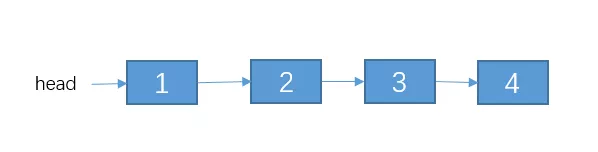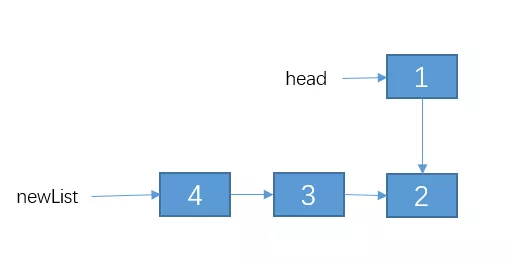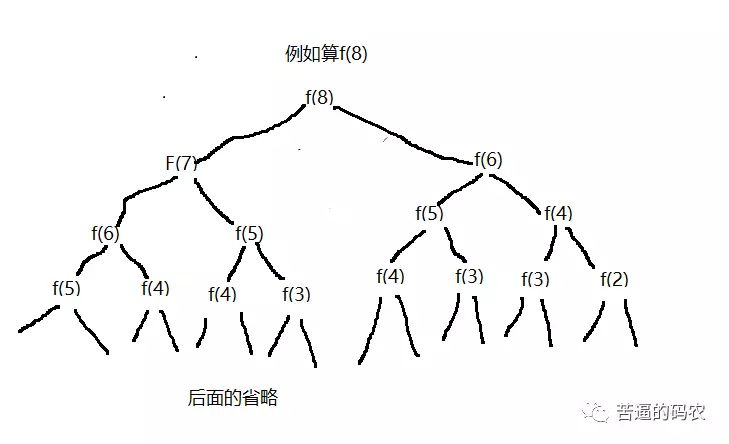# 告别递归算法，谈谈我的一些经验

#### 递归的三大要素

// 算 n 的阶乘(假设n不为0)
int f(int n){

}


// 算 n 的阶乘(假设n不为0)
int f(int n){
if(n == 1){
return 1;
}
}


// 算 n 的阶乘(假设n>=2)
int f(int n){
if(n == 2){
return 2;
}
}


// 算 n 的阶乘(假设n不为0)
int f(int n){
if(n <= 2){
return n;
}
}


f(n) = n * f(n-1)。

// 算 n 的阶乘(假设n不为0)
int f(int n){
if(n <= 2){
return n;
}
// 把 f(n) 的等价操作写进去
return f(n-1) * n;
}


#### 案例1：斐波那契数列

1、第一递归函数功能

int f(int n){

}


2、找出递归结束的条件

int f(int n){
if(n <= 2){
return 1;
}
}


int f(int n){
// 1.先写递归结束条件
if(n <= 2){
return 1;
}
// 2.接着写等价关系式
return f(n-1) + f(n - 2);
}


#### 案例2：小青蛙跳台阶

1、第一递归函数功能

int f(int n){

}


2、找出递归结束的条件

int f(int n){
if(n == 1){
return 1;
}
}


int f(int n){
if(n == 1){
return 1;
}
ruturn f(n-1) + f(n-2);
}


int f(int n){
//f(0) = 0,f(1) = 1，等价于 n<=2时，f(n) = n。
if(n <= 2){
return n;
}
ruturn f(n-1) + f(n-2);
}


#### 案例3：反转单链表。

class Node{
int date;
Node next;
}


1、定义递归函数功能

Node reverseList(Node head){

}


2. 寻找结束条件

Node reverseList(Node head){
}
}


3. 寻找等价关系Node reverseList(Node head){
}
// 我们先把递归的结果保存起来，先不返回，因为我们还不清楚这样递归是对还是错。，
}//用递归的方法反转链表
// 1.递归结束条件
}
// 递归反转 子链表
// 改变 1，2节点的指向。
// 让 2 的 next 指向 1
// 1 的 next 指向 null.
// 把调整之后的链表返回。
return newList;
}


#### 有关递归的一些优化思路

1. 考虑是否重复计算// 我们实现假定 arr 数组已经初始化好的了。
int f(int n){
if(n <= 2){
return n;
}
//先判断有没计算过
if(arr[n] != -1){
//计算过，直接返回
return arr[n];
}else{
// 没有计算过，递归计算,并且把结果保存到 arr数组里
arr[n] = f(n-1) + f(n-2);
reutrn arr[n];
}
}


2. 考虑是否可以自底向上

f(1) = 1;

f(2) = 2;

public int f(int n) {
if(n <= 2)
return n;
int f1 = 1;
int f2 = 2;
int sum = 0;

for (int i = 3; i <= n; i++) {
sum = f1 + f2;
f1 = f2;
f2 = sum;
}
return sum;
}


### 评论(19)

• mpweixin用户 普通 2022-01-15 11:24 上午

请问下帅地能不能讲下链表逆置的部分，原先每个节点的next指针是实现如何逆置的。我愚笨，是真的想了半天不明白，希望有空能回复下，十分感谢🙏

• li~ 普通 2021-09-30 3:00 下午

讲得不错，易懂

• Copera~ 普通 2021-09-26 11:28 上午

讲的不错，支持了

• young 普通 2021-09-13 12:56 下午

shuidi要坚持下去呀

• 帅地 永久会员 @ young 2021-09-13 2:41 下午

一定滴，不过最近秋招，在更面试题哦，秋招过后，会把算法这些专题补上来。

• Rey 普通 2021-07-05 8:57 下午

写的真不错

• 田山杉 普通 2021-06-30 4:26 下午

分析到位，易懂！关键字 return 拼写手误！

• 绿樱 普通 2021-06-28 9:32 上午

“有关递归的一些优化思路”里的第一个代码片段里面arr[n] = f(n-1) + f(n-1);应该是arr[n] = f(n-1) + f(n-2);吧

• 星归 普通 2021-05-12 9:12 上午

• Elaine 2021-05-11 11:11 下午

师兄反转链表的讲得好好 get到了新思路！

• Windfloat 普通 2021-05-11 11:19 上午

五大算法思想。。期待

• 梅花五瓣 2021-04-28 11:03 上午

思路超清晰，写得真好

• 怀独无傲 普通 2021-04-22 11:07 上午

1